# Horizontal Boxplots with Points using Seaborn in Python

• Last Updated : 26 Nov, 2020

Seaborn is an amazing visualization library for statistical graphics plotting in Python. It provides beautiful default styles and color palettes to make statistical plots more attractive. It is built on the top of matplotlib library and also closely integrated into the data structures from pandas.
Seaborn aims to make visualization of the central part of exploring and understanding data. It provides dataset-oriented APIs, so that we can switch between different visual representations for the same variables for a better understanding of the dataset.

## Why horizontal Boxplots will be used instead of boxplots?

They are useful when you have numerous groups with larger names. Boxplots works fine when we have a limited number of groups and short names of groups. But if we have longer titles they would overlap each other in boxplots, and it will get difficult to read the data and taking insights from the graph. Horizontal boxplots solve that problem very easily.

Attention geek! Strengthen your foundations with the Python Programming Foundation Course and learn the basics.

To begin with, your interview preparations Enhance your Data Structures concepts with the Python DS Course. And to begin with your Machine Learning Journey, join the Machine Learning - Basic Level Course

### Below is the step-by-step Approach to illustrate Horizontal Boxplots using Seaborn module:

• Import libraries

## Python3

 `# Import required modules``import` `sklearn``import` `numpy as np``import` `matplotlib.pyplot as plt``import` `pandas as pd``import` `seaborn as sns`
• Assign data

## Python3

 `# To avoid changing values everytime you run the cell``np.random.seed(``42``)`` ` `# Creating Data``df ``=` `pd.DataFrame({``    ``'Ice-cream'``: np.random.normal(``40``, ``15``, ``100``),``    ``'Chocolate'``: np.random.normal(``60``, ``10``, ``100``),``    ``'Cakes'``: np.random.normal(``80``, ``5``, ``100``)``})`` ` `# Display data``print``(df)`

Output: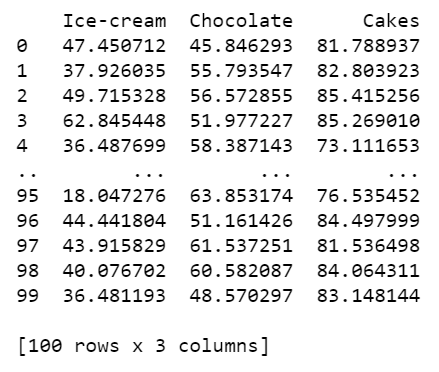Mostly data we come across are in wide format. For example when you scrape a website. This data is in the wide form Convert the data into long-form by using pandas melt function.

## Python3

 `# Using melt dataframe for Converting data to long form``data_df ``=` `df.melt(var_name``=``'Dessert'``, value_name``=``'Votes'``)`` ` `# Data is in long form``print``(data_df.head())`

Output: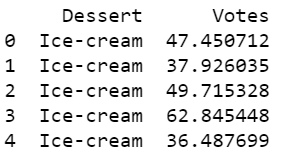• Plot this data into a boxplot.

## Python3

 `# Adjust size``plt.figure(figsize``=``(``8.3``,``6``))`` ` `# Illustrate boxplot``sns.boxplot(y``=``"Dessert"``, x``=``"Votes"``, data``=``data_df)`

Output: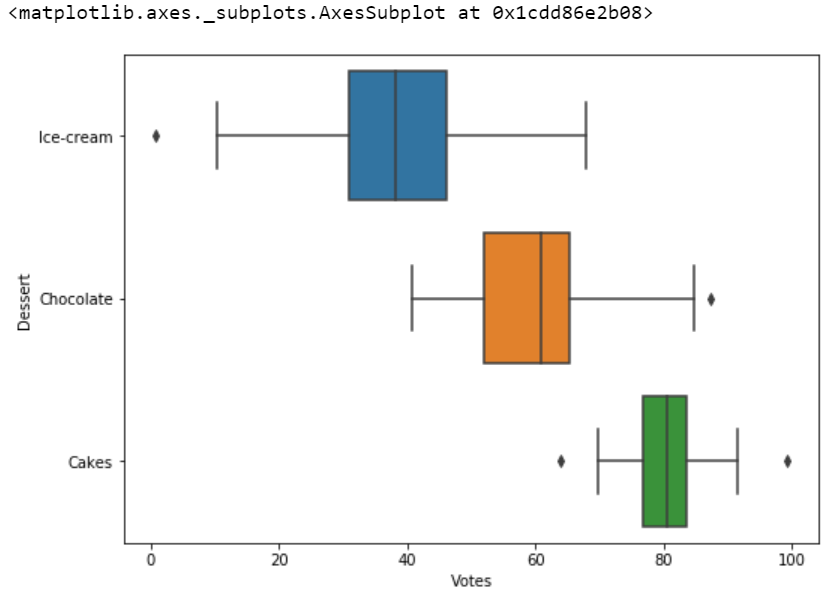After plotting the data points into the boxplot. Strip plot can also be drawn on its own but it will complement while using with boxplot or violin plot.

## Python3

 `# It will create the data points inside the boxplot``# Illustrating box plot``sns.boxplot(y``=``"Dessert"``, x``=``"Votes"``, data``=``data_df)`` ` `# Illustrating strip plot``sns.stripplot(y``=``"Dessert"``, x``=``"Votes"``, color``=``'black'``,``              ``alpha``=``0.3``, data``=``data_df)`

Output: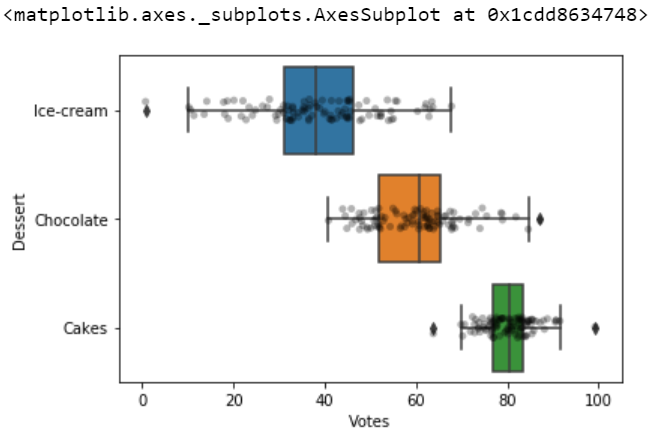Changing our boxplot into the horizontal boxplot with data points by simply swapping the axes when we have numerous columns then names overlap with each other, so then we use a horizontal boxplot.

## Python3

 `# You can simply change into horizontal ``# boxplots by swapping  x and y axes.``# Illustrating box plot``sns.boxplot(x``=``"Dessert"``, y``=``"Votes"``, data``=``data_df)`` ` `# Illustrating strip plot``sns.stripplot(x``=``"Dessert"``, y``=``"Votes"``, color``=``'black'``,``              ``alpha``=``0.3``, data``=``data_df)`

Output: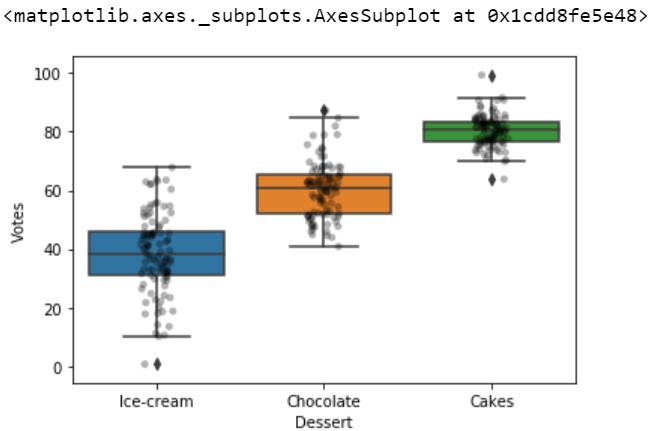Below is the complete program based on the above approach:

## Python3

 `# Import required modules``import` `sklearn``import` `numpy as np``import` `matplotlib.pyplot as plt``import` `pandas as pd``import` `seaborn as sns`` ` ` ` `# To avoid changing values everytime you run the cell``np.random.seed(``42``)`` ` ` ` `# Creating Data``df ``=` `pd.DataFrame({``    ``'Ice-cream'``: np.random.normal(``40``, ``15``, ``100``),``    ``'Chocolate'``: np.random.normal(``60``, ``10``, ``100``),``    ``'Cakes'``: np.random.normal(``80``, ``5``, ``100``)``})`` ` ` ` `# Using melt dataframe for Converting data to long form``data_df ``=` `df.melt(var_name``=``'Dessert'``, value_name``=``'Votes'``)`` ` ` ` `# Adjust size``plt.figure(figsize``=``(``8.3``, ``6``))`` ` ` ` `# Assign title``plt.title(``'Horizontal Boxplots with Points using Seaborn'``)`` ` ` ` `# Illustrating box plot``sns.boxplot(y``=``"Dessert"``, x``=``"Votes"``, data``=``data_df)`

Output: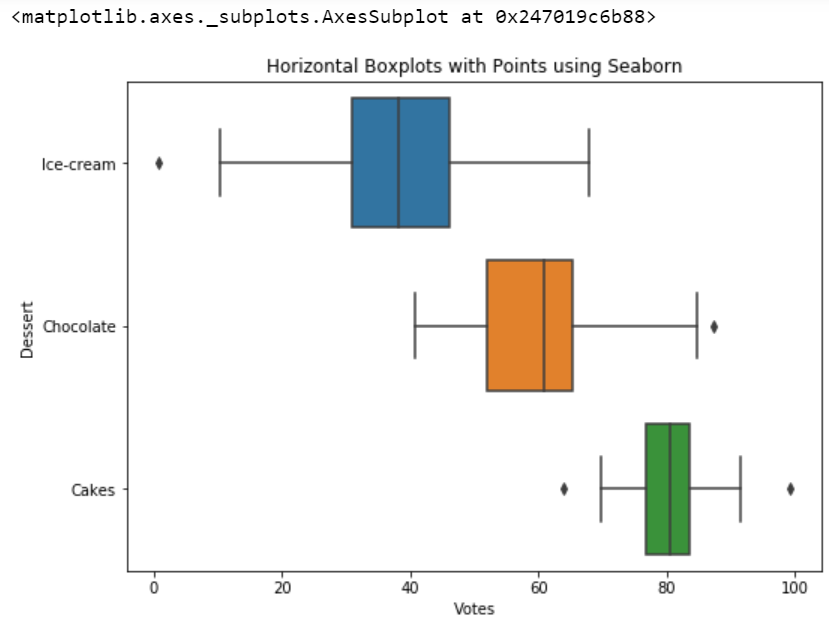My Personal Notes arrow_drop_up